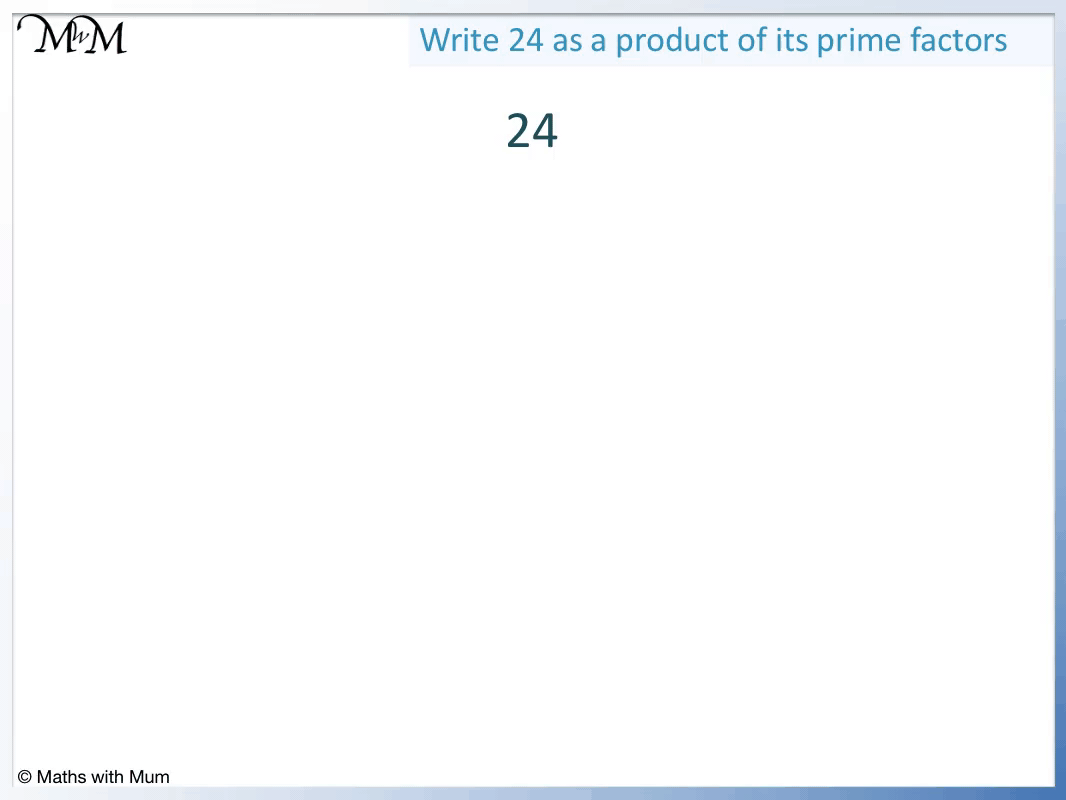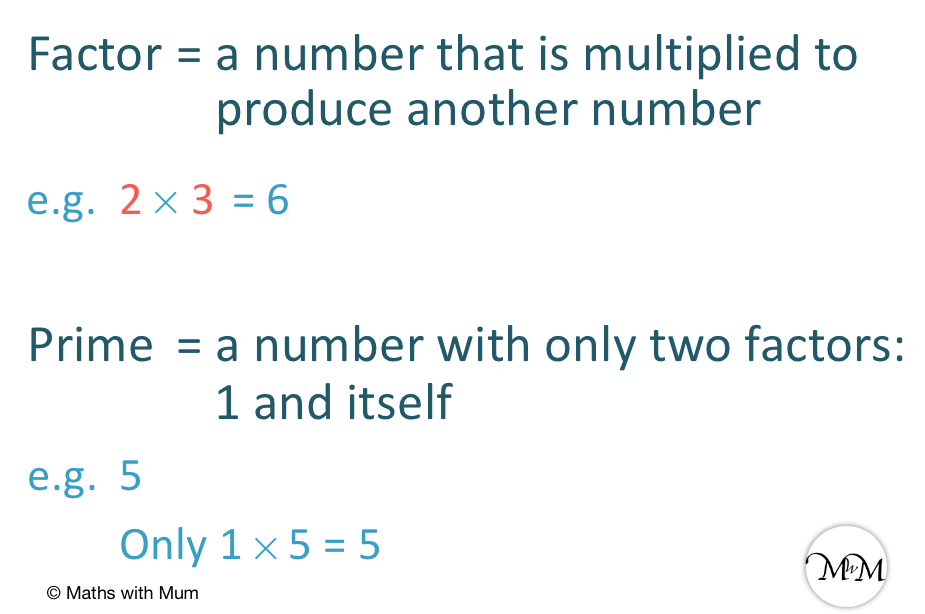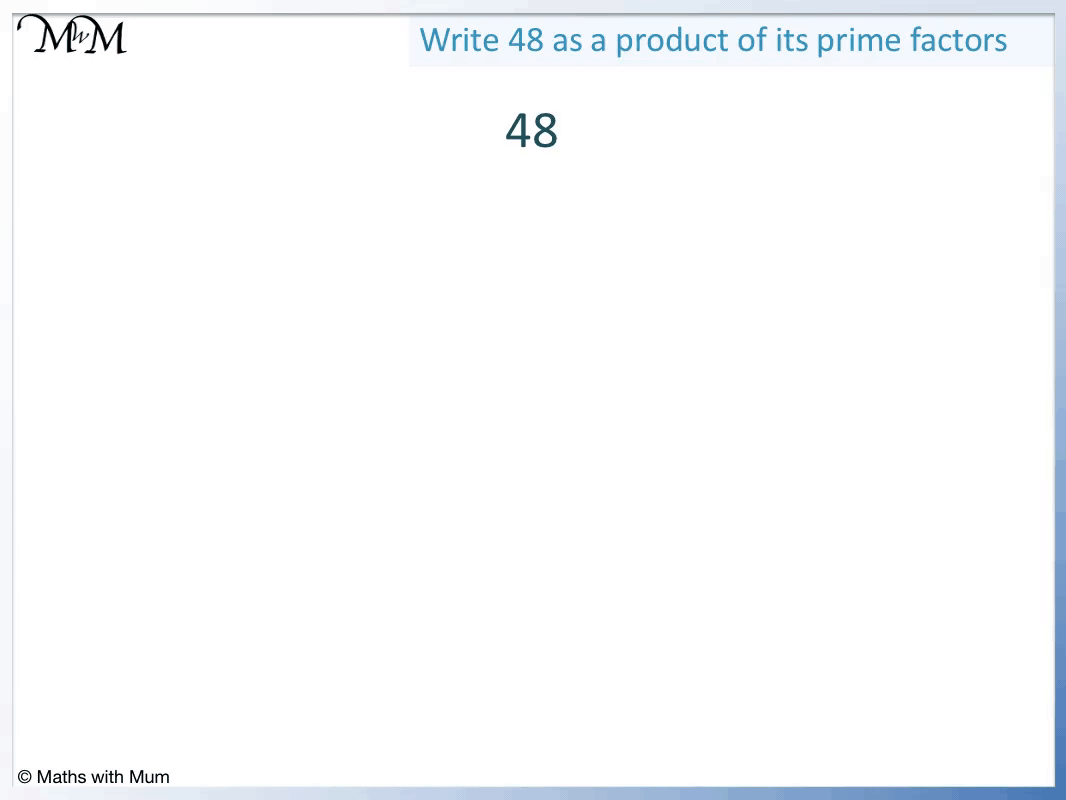# Prime Factor Trees

Prime Factor Trees• A factor tree is a method used to write a number as the product of its prime factors.
• This means finding the prime numbers that multiply together to make that number.
• A factor is a number that divides exactly into another number
• A prime number is a number that has only 2 factors: 1 and itself.
• For each number in the tree, find a pair of whole numbers that multiply to make that number.
• Every time we reach a prime number, we stop and then circle that number.
• 24 = 12 x 2.
• 2 is prime because it can only be made by 1 x 2. We circle it.
• We never choose 1 as a factor in our factor trees because we would end up with the same number again and 1 is not primema.
• 12 can then be written as 2 x 6.
• Again, 2 is prime, so we circle it.
• 6 can be written as 2 x 3.
• Both 2 and 3 are prime, so we circle them.
• We write 24 as the circled prime numbers multiplied togther.
• The prime factorisation of 24 is 24 = 2 x 2 x 2 x 3.
• We can write this prime factorisation in index form as 24 = 23 x 3.
A prime factor tree is a diagram used to find the prime numbers that multiply to make the original number.• 36 can be written as 9 x 4.
• 9 can be written as 3 x 3.
• 3 is prime because it cannot be divided to make a smaller whole number. We circle the threes.
• 4 can be written as 2 x 2.
• 2 is prime because it cannot be divided to make a smaller whole number. We circle the twos.
• The prime factorisation of 36 is 36 = 2 x 2 x 3 x 3.
• We can write this in index form as 36 = 22 x 32.
• It does not matter which numbers were chosen in each stage of the factor tree, the final prime factorisation will always be the same.Supporting Lessons# Prime Factor Trees

## What are Prime Factor Trees

Prime factor trees are diagrams that show the prime factorisation of a number. The number is broken down into two of its factors. These factors are then broken down into their factors until only prime numbers remain.

A factor is a number that divides exactly into another number. Two factors can multiply to make another number.

A prime number is a number that has only two factors, which are 1 and the number itself. Put simply, a prime number cannot be divided by a whole number to make a smaller whole number.Here is an example of a prime factor tree for the number 36.

A factor tree is a diagram which helps us to find the prime numbers that can multiply together to make the original number.We first think of two numbers that multiply to make 36.

36 = 9 × 4 and so, we draw two lines coming out of 36 with 9 and 4 at the ends of them. We say that 9 and 4 are factors of 36.

We can continue to split these numbers into smaller factors.

9 can be written as 3 × 3 and 4 can be written as 2 × 2.

Now we circle the threes and the twos because they are prime. We know this because the only way to make 3 by multiplying whole numbers is 1 × 3 and the only way to make 2 by multiplying whole numbers is 1 × 2.

We write 36 as these circled prime numbers multiplied together.

36 = 2 × 2 × 3 × 3.

We can check this by multiplying the numbers. 2 × 2 = 4 and 3 × 3 = 9. 4 × 9 = 36.

## Common Mistakes

A common mistake when drawing factor trees is to write a prime number as 1 × itself. We do not include 1 in the prime factor tree because 1 is technically not a prime number. It also does not help us because we just get the same number appearing again.

Another common mistake is to find two numbers that add to make the number above it instead of numbers that multiply to make the number above it.

## How to do Prime Factor Trees

To do prime factor trees, follow these steps:

1. Draw two diagonal lines below the number.
2. At the ends of the two lines, write two numbers that multiply to make the number above the lines.
3. Repeat steps 1 and 2 for each new number written unless it is a prime number.
4. If the number is a prime number, circle it.
5. When all numbers at the base of the tree have been circled, the method is complete.
6. Write the original number as the product of the circled prime numbers.

For example, here is a prime factor tree for the number 24.

The first step is to draw two diagonal lines below the number.

The second step is to write two numbers that multiply to make 24. 12 × 2 = 24 and so, we write 12 and 2 at the end of each line.We now repeat these steps for each factor as long as it is not a prime number.

2 is a prime number because it cannot be broken down into any smaller whole numbers. We circle it.

We draw lines below 12 and write it as 6 × 2. Again, 2 is prime so we circle it.

6 can be written as 2 × 3. Both 2 and 3 are prime and so we circle them both.

We write the original number as the product of the circled prime numbers.

24 = 2 × 2 × 2 × 3.

It does not matter which numbers are chosen at each stage of the factor tree. The final prime factorisation will always be the same.

For example, we could write 24 as 4 × 6. We can then write 4 as 2 × 2 and 6 as 2 × 3.

We still arrive at 24 = 2 × 2 × 2 × 3.

## How to Write the Prime Factorisation of a Number

To write the prime factorisation of a number, follow these steps:

1. Write the number as the product of any two of its factors.
2. If the factors are prime, circle them.
3. If a factor is not prime, write it as the product of any two of its factors.
4. Repeat steps 2 and 3 until all new factors written are prime and there are no more factors to find.
5. The prime factorisation of the number is written as all of the circled prime numbers multiplied together.

For example, here is the prime factorisation of 48.We write 48 as 6 × 8.

We write 6 as 3 × 2 and we write 8 as 4 × 2. 2 and 3 are prime so we can circle these numbers.

We can write 4 as 2 × 2, which again can be circled because they are prime.

To write the prime factorisation of a number, write the prime numbers multiplied together.

48 = 2 × 2 × 2 × 2 × 3.

We can write numbers that have been factorised in index form.

2 × 2 × 2 × 2 = 24 because 4 twos are multiplied together.

We can write 48 = 24 × 3.

It does not matter which numbers are chosen at each stage of the prime factor tree, the prime factorisation of a number is always the same.

Prime numbers are the building blocks of all other numbers and the prime factorisation of a number is unique to that number.Now try our lesson on What are Square Numbers where we learn what square numbers are.